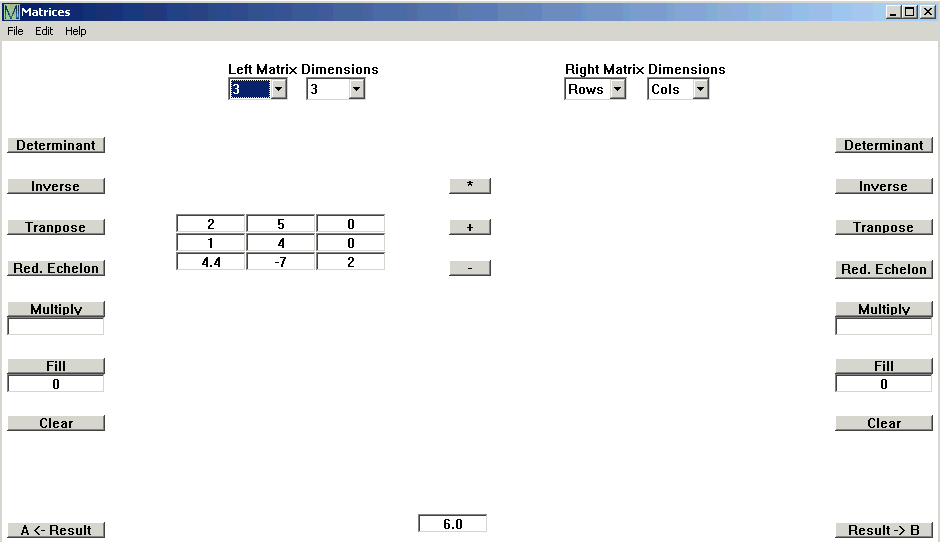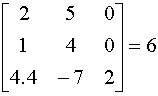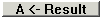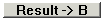# Determinant

Once you have entered your matrix, click on Determinant. The result appears at the centre bottom of the window, like this.Here the determinant ofThere are actually two Determinant buttons; the left one works on the Left Matrix; the right one on the Right Matrix. In either case the determinant, the result, is at the bottom of the window.

Determinant is only defined if the matrix is a square. Determinant will not  work when the number of rows is not equal to the number of columns.

If you want to work with this number, click the buttonThe Left Matrix disappears and is replaced by the number.

You can also place the answer in the Right Matrix by clicking